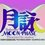# Regarding the concept of "Twice the Speed of Light"

Let the speed of light = c

If 2 object travels in the opposite direction at the speed of light.

Is it suffice for me to say that the distance generated by these 2 object is at the rate of 2c?

I am not here questioning whether or not an object can travel faster than speed of light, but rather on instances where "something" may increase or decrease faster than the speed of light. (Rate of change)

In this case here, I am referring to distance generated by 2 object traveling in opposite direction.

I am only a layman, If it is unacceptable to declare anything traveling at/near speed of c, I am fine with 0.51c or something as long as when both add together, it is greater than c.

I know this may be a redundant post that was raised millions of times but I just couldn't find the right keyword that can derive me the answer. Most of the time I get search results regarding arguments of no object can travel faster than speed of light when what I want to know is not related to object but the rate of change.Note by Ne-ko Nya
6 months, 2 weeks ago

This discussion board is a place to discuss our Daily Challenges and the math and science related to those challenges. Explanations are more than just a solution — they should explain the steps and thinking strategies that you used to obtain the solution. Comments should further the discussion of math and science.

When posting on Brilliant:

• Use the emojis to react to an explanation, whether you're congratulating a job well done , or just really confused .
• Ask specific questions about the challenge or the steps in somebody's explanation. Well-posed questions can add a lot to the discussion, but posting "I don't understand!" doesn't help anyone.
• Try to contribute something new to the discussion, whether it is an extension, generalization or other idea related to the challenge.

MarkdownAppears as
*italics* or _italics_ italics
**bold** or __bold__ bold
- bulleted- list
• bulleted
• list
1. numbered2. list
1. numbered
2. list
Note: you must add a full line of space before and after lists for them to show up correctly
paragraph 1paragraph 2

paragraph 1

paragraph 2

[example link](https://brilliant.org)example link
> This is a quote
This is a quote
    # I indented these lines
# 4 spaces, and now they show
# up as a code block.

print "hello world"
# I indented these lines
# 4 spaces, and now they show
# up as a code block.

print "hello world"
MathAppears as
Remember to wrap math in $$ ... $$ or $ ... $ to ensure proper formatting.
2 \times 3 $2 \times 3$
2^{34} $2^{34}$
a_{i-1} $a_{i-1}$
\frac{2}{3} $\frac{2}{3}$
\sqrt{2} $\sqrt{2}$
\sum_{i=1}^3 $\sum_{i=1}^3$
\sin \theta $\sin \theta$
\boxed{123} $\boxed{123}$

Sort by:

Have a look at https://en.wikipedia.org/wiki/Faster-than-light particularly the section on closing speeds.

- 6 months, 2 weeks ago

Is it suffice for me to say that the distance generated by these 2 object is at the rate of 2c?

My knowledge of relativity is not good enough to answer that question, but I think length contraction could have an influence on the result.

A length measured by an observer moving with respect to a stationary observer may be measured to be shorter in the frame of the stationary observer.

- 6 months, 2 weeks ago